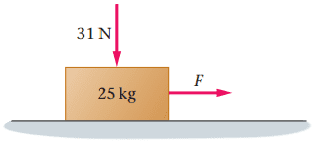# How to find the minimum horizontal force?

## Homework Statement

What is the minimum horizontal force F needed to make the box start moving in (Figure 1) ? The coefficients of kinetic and static friction between the box and the floor are 0.28 and 0.39, respectively.fk=mu N
fs=mu N

## The Attempt at a Solution

Im not sure that the N pointing downward is the normal force or not because I though normal force = 25kg* 9.81m/s2=245?

so would the minimum force be Fs= mu N......... (.39)(245)=96?
i just don't know what the 31 N signifies since it is pointing downward??? help please
[/B]

It looks to me like you have a block of mass 25 kg (which, of course, has weight) and an additional force of 31 Newtons acting downward on the block.

The equation you wrote for the normal force would be correct for a block of 25 kg sitting on a flat surface with no other forces acting on it.

okay, how would I incorporate that extra force of 31 N?

would I multiply the 31 N by the static friction? 31 N *36 N?

I would say to draw a free body diagram with all of the forces - weight of block, 31 N force downward and the normal force N upward. Since there is no acceleration in the y direction (or the x direction for that matter) the block is in equilibrium so the forces should sum (rather, have to sum) to equal 0.

When the block is just about ready to slide, as you know, friction force Ff = μsN. So you have to find the normal force N.

Fs= .39 * (-31N) + 245(weight) ?

am I correct?

So to start with lets consider a stationary block of 25kg sitting on the floor, it's not moving, it's not sinking into the ground so the weight of the block must be balanced by the normal reaction force: W = 245N = Normal Reaction Force (Nf)
Now we have an extra force of 31N pushing down on the block, yet the block is still not sinking/merging into the ground, therefore the normal reaction force must again be accounting for this extra force: Nf = weight + extra downward force = 245 + 31 = 276N
As discussed above when the vlock is about to slide: Ff = μsNf
Sub you values in, what do you get?

Forget about the friction force for now. Before you can find the friction force, you have to find the normal force. Once you find the normal force, then you can calculate the friction force. To find the normal force, you have to sum all of the vertical components of force.

Let's say I have a crate sitting on a scale and the scale reads 100 pounds. Now let's say I throw 5 more 10-pound boxes on top of that crate. What is the normal force acting on the crate? I will give you a hint: The scale knows the answer. That is the same situation you have in your problem. You have a block that has weight and you are adding more weight on top of it.

•Ff=(.39)* 276= 108 N
why are we adding the downward force and not subtracting it?

Forget about the friction force for now. Before you can find the friction force, you have to find the normal force. Once you find the normal force, then you can calculate the friction force. To find the normal force, you have to sum all of the vertical components of force.

Let's say I have a crate sitting on a scale and the scale reads 100 pounds. Now let's say I throw 5 more 10-pound boxes on top of that crate. What is the normal force acting on the crate? I will give you a hint: The scale knows the answer. That is the same situation you have in your problem. You have a block that has weight and you are adding more weight on top of it.

makes sense! thankyou

•TomHart
The downward force is pushing the block into the ground. But the block isn't sinking into the ground, it isn't moving. Hence there is no unbalanced force. If there is no unbalanced force there must be a force (in this case the normal reaction force) counter-acting it.

•Angela_vaal